Question

# Suppose a 250. mL flask is filled with 1.2 mol of Cl₂

Suppose a 250. mL flask is filled with 1.2 mol of Cl₂, 1.8 mol of CHCl₃ and 0.90 mol of HCl. The following reaction becomes possible:

Cl₂(g)+CHCl₃(g) ⇌ HCl(g)+CCl₄(g)

The equilibrium constant K for this reaction is 0.855 at the temperature of the flask.

Calculate the equilibrium molarity of HCl. Round your answer to two decimal places.

Initial concentration of Cl2 = mol of Cl2 / volume in L
= 1.2 mol / 0.250 L
= 4.8 M

Initial concentration of CHCl3 = mol of CHCl3 / volume in L
= 1.8 mol / 0.250 L
= 7.2 M

Initial concentration of HCl = mol of HCl / volume in L
= 0.90 mol / 0.250 L
= 3.6 M

ICE Table: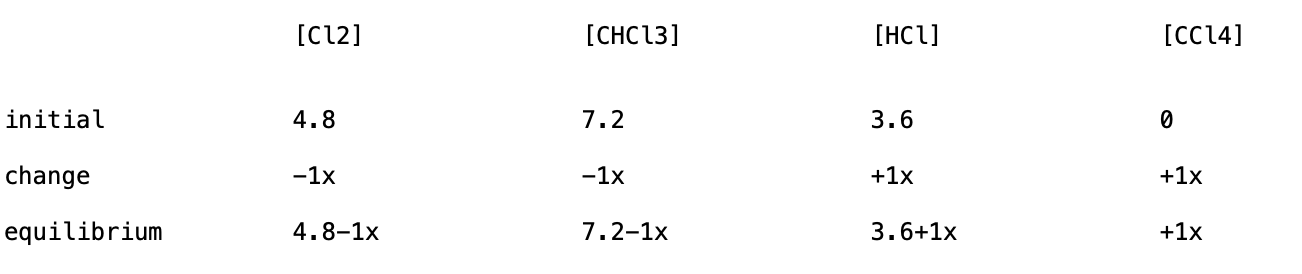Equilibrium constant expression is
Kc = [HCl]*[CCl4]/[Cl2]*[CHCl3]
0.855 = (3.6 + 1*x)(1*x)/((4.8-1*x)(7.2-1*x))
0.855 = (3.6*x + 1*x^2)/(34.56-12*x + 1*x^2)
29.55-10.26*x + 0.855*x^2 = 3.6*x + 1*x^2
29.55-13.86*x-0.145*x^2 = 0
a = -0.145
b = -13.86
c = 29.55

Roots can be found by
x = {-b + sqrt(b^2-4*a*c)}/2a
x = {-b - sqrt(b^2-4*a*c)}/2a

b^2-4*a*c = 2.092*10^2

roots are :
x = -97.67 and x = 2.086

since x can't be negative, the possible value of x is
x = 2.086

At equilibrium:
[HCl] = 3.6+1x = 3.6+1*2.086 = 5.69 M

#### Earn Coins

Coins can be redeemed for fabulous gifts.

Similar Homework Help Questions
• ### Suppose a 250. mL flask is filled with 2.0 mol of Cl, 0.20 mol of CHCl,...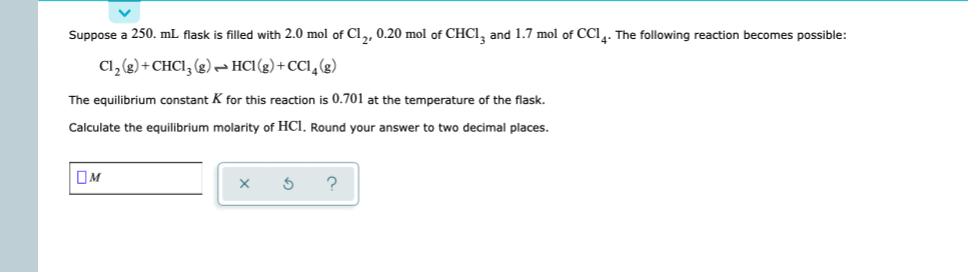Suppose a 250. mL flask is filled with 2.0 mol of Cl, 0.20 mol of CHCl, and 1.7 mol of CCI4. The following reaction becomes possible: Cl2(g) + CHCI, (8) HCI(g) +CCI (8) The equilibrium constant K for this reaction is 0.701 at the temperature of the flask. Calculate the equilibrium molarity of HCl. Round your answer to two decimal places. OM 1 x s ?

• ### Suppose a 500. ml flask is filled with 1.2 mol of Cl, and 0.80 mol of...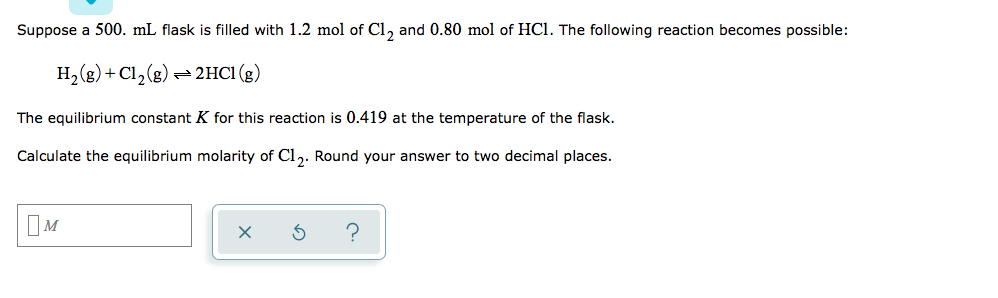Suppose a 500. ml flask is filled with 1.2 mol of Cl, and 0.80 mol of HCl. The following reaction becomes possible: H2(g) + Cl2(g) = 2HCl (g) The equilibrium constant K for this reaction is 0.419 at the temperature of the flask. Calculate the equilibrium molarity of Cl. Round your answer to two decimal places. xs ?

• ### Suppose a 250. ml flask is filled with 1.5 mol of Cl, and 1.3 mol of...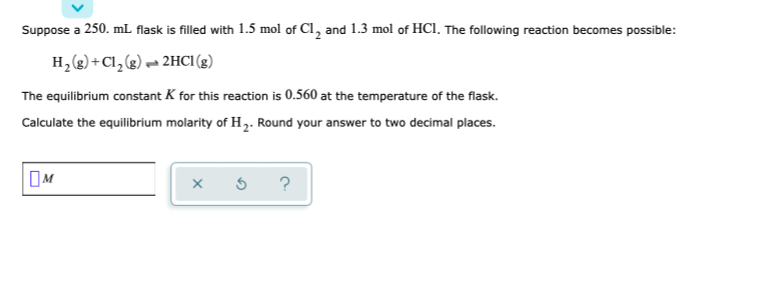Suppose a 250. ml flask is filled with 1.5 mol of Cl, and 1.3 mol of HCl. The following reaction becomes possible: H2(g) + Cl2(g) 2HCl (8) The equilibrium constant K for this reaction is 0.560 at the temperature of the flask. Calculate the equilibrium molarity of H. Round your answer to two decimal places. OM * 5 ?

• ### Suppose a 250. mL. flask is filled with 1.8 mol of Cl2 and 0.60 mol of...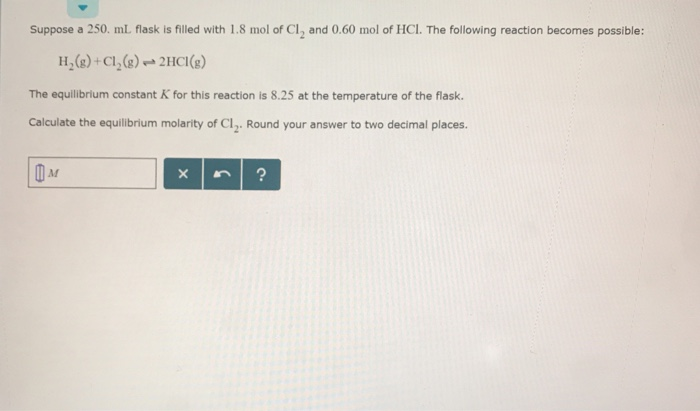Suppose a 250. mL. flask is filled with 1.8 mol of Cl2 and 0.60 mol of HCl. The following reaction becomes possible: H(+Cl()2HCI) The equilibrium constant K for this reaction is 8.25 at the temperature of the flask. Calculate the equilibrium molarity of Cl2. Round your answer to two decimal places.

• ### Suppose a 250. ml flask is filled with 1.6 mol of Cl, and 1.3 mol of...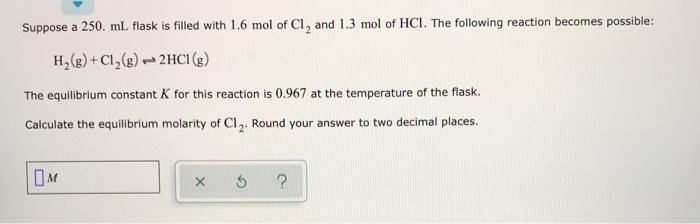Suppose a 250. ml flask is filled with 1.6 mol of Cl, and 1.3 mol of HCI. The following reaction becomes possible: H2(g) + Cl2(g) → 2HCI (g) The equilibrium constant K for this reaction is 0.967 at the temperature of the flask. Calculate the equilibrium molarity of C1. Round your answer to two decimal places. OM I ?

• ### Suppose a 250. ml flask is filled with 0.50 mol of H, and 0.40 mol of...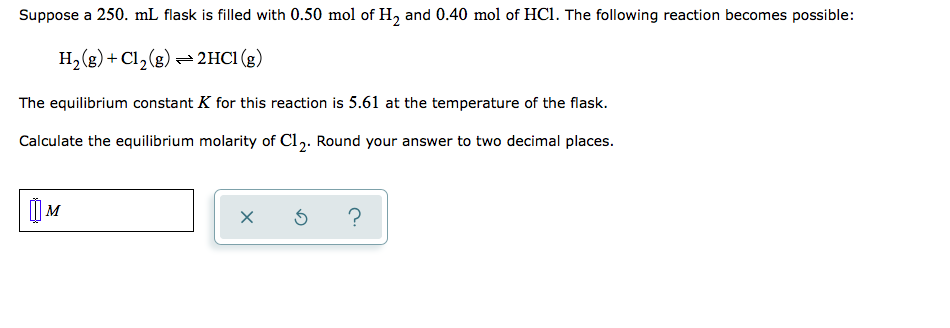Suppose a 250. ml flask is filled with 0.50 mol of H, and 0.40 mol of HCl. The following reaction becomes possible: H2(g) + Cl2(g) = 2HCl (g) The equilibrium constant K for this reaction is 5.61 at the temperature of the flask. Calculate the equilibrium molarity of Cl. Round your answer to two decimal places. TOM xo?

• ### Suppose a 250. mL flask is filled with 1.8 mol of No₃ and 1.5 mol of NO₂.

Suppose a 250. mL flask is filled with 1.8 mol of No₃ and 1.5 mol of NO₂. The following reaction becomes possible: No₃(g) + No(g) ⇄ 2No₂(g) The equilibrium constant K for this reaction is 3.20 at the temperature of the flask. Calculate the equilibrium molarity of No₂. Round your answer to two decimal places.

• ### Suppose a 500. ml flask is filled with 1.7 mol of Cl, and 2.0 mol of...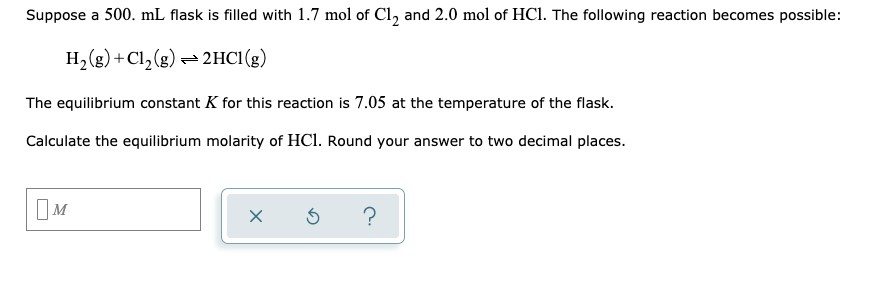Suppose a 500. ml flask is filled with 1.7 mol of Cl, and 2.0 mol of HCl. The following reaction becomes possible: H2(g) +C12(g) + 2HCl (8) The equilibrium constant K for this reaction is 7.05 at the temperature of the flask. Calculate the equilibrium molarity of HCl. Round your answer to two decimal places. x o ?

• ### Suppose a 500. mL flask is filled with 1.0 mol of CHCl, 1.6 mol of Hcl...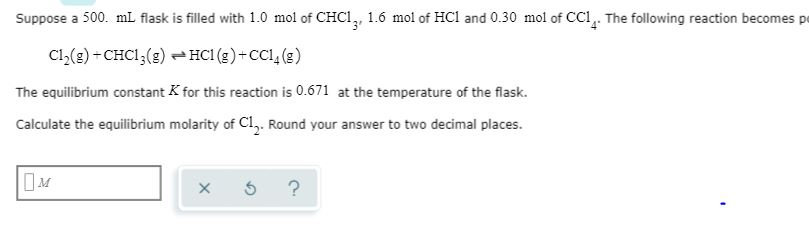Suppose a 500. mL flask is filled with 1.0 mol of CHCl, 1.6 mol of Hcl and 0.30 mol of CCI,. The following reaction becomes p Cl(g)+CHCI (g) HCI (s)+CC () The equilibrium constant K for this reaction is 0.671 at the temperature of the flask. Calculate the equilibrium molarity of Cl,. Round your answer to two decimal places. ?

• ### Suppose a 500 ml flask is filled with 1.2 mol of NO, and 0.40 mol of...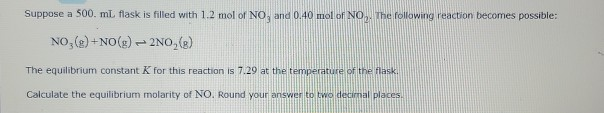Suppose a 500 ml flask is filled with 1.2 mol of NO, and 0.40 mol of NO the following reaction becomes possible: NO,() +NO(g) - 2NO(g) The equilibrium constant K for this reaction is 7.29 at the temperature of the flask Calculate the equilibrium molarity of NO. Round your answer to two decimal places